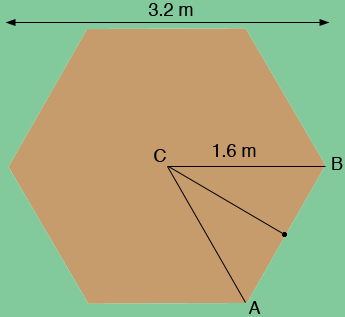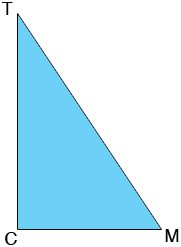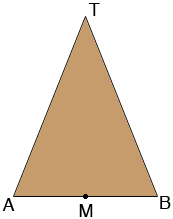SEARCH HOMEMath Central Quandaries & QueriesQuestion from mike, a parent: we are thinking about making a lightweight tipi tent but we need to know what the angles and lengths of each side that we will need to cut. The height of the tipi wants to be about 2.2m span at the widest point wants to be about 3.2m we want to make it based upon a 6 sided(hexagon) shape thanks for your helpHi Mike,

If the base is a regular hexagon then The angle BCA below measures 360/6 = 60 degrees.Since the length of BC and CA are equal the angles ABC and CAB are equal and hence also measure 60 degrees. Thus triangle BCA is an equilateral triangle and the length of AB is 1.1 m.

In the diagram above triangle M is the midpoint of AB and triangle BCM is a right triangle so Pythagoras theorem gives

|CM|2 + |MB|2 = |BC|2

and thus

|CM| = √(1.62 - 0.82) = 1.39 m.

Now look at the triangle TCM where T is the peak of the tipi.This is also a right triangle and using Pythagoras theorem again gives

|TM| = √(2.22 + 1.392) = 2.6 m.

For each of the 6 sides of the tipi I would measure a base of 1.6 m and from the midpoint M of the base measure upwards 2.6 m to T. Check that the lengths of TA and BT are equal.In the central plains of Canada where Math Central is located the Indigenous people lived in tipis. On a companion web site to Math Central called Aboriginal Perspectives there are video clips of Aboriginal leaders teaching children to raise a tipi in traditional fashions. You can see the clips here and here.

HarleyMath Central is supported by the University of Regina and The Pacific Institute for the Mathematical Sciences.